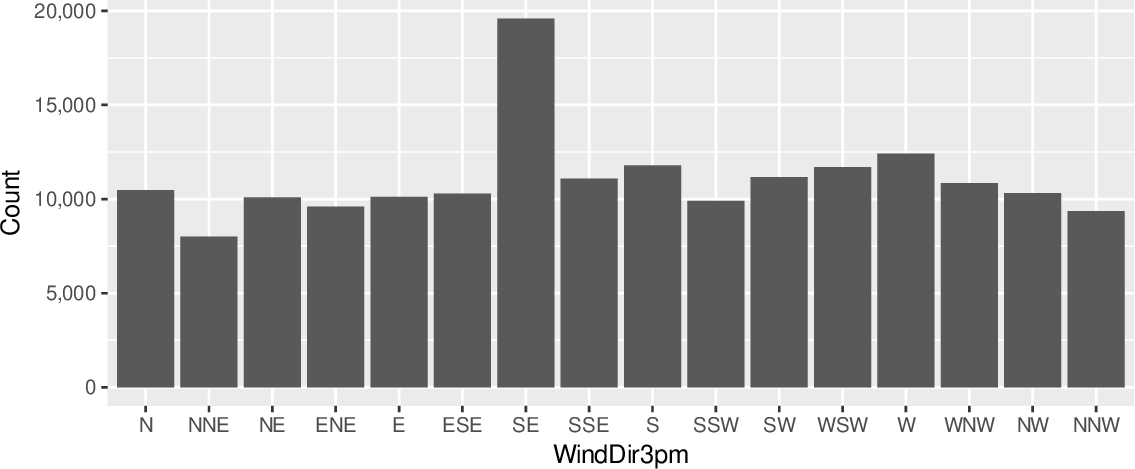Data Science Desktop Survival Guide by Graham WilliamsDesktop Survival Project Home Preface Data Science Introducing R R Constructs R Tasks R Strings R Read, Write, and Create Data Template Data Exploration Data Wrangling Data Visualisation Statistics ML Template ML Scenarios ML Activities ML Applications ML Algorithms Cluster Analysis Decision Trees Computer Vision Graph Data Privacy Literate Data Science Coding with Style Resources Bibliography Index

## Bar Chart Basic

20200427ds %>%   ggplot(aes(x=wind_dir_3pm)) +   geom_bar() +   scale_y_continuous(labels=comma) +   labs(x=vnames["wind_dir_3pm"], y="Count") A common and simple plot is the bar chart which displays bars with a height that corresponds to the number of observations having that value of the variable displayed on the x-axis. We use ggplot2::geom_bar() to add a bar chart layer to a plot. Only an x-axis aesthetic is required using ggplot2::aes() with the x= option. The y-axis is automatically computed. In our example we pipe the dataset on to ggplot2::ggplot(), specifying the x-axis as the categoric variable wind_dir_3pm (the x-axis). The count is automatically determined from the dataset by ggplot2::geom_bar() which then adds the layer of bars to the plot. The y-ticks use commas with ggplot2::scale_y_continuous() using the labels=comma option. It is crucial that for large numbers commas separate the thousands, so that the reader is able to easily read the number. There can be catastrophic outcomes from a misreading of numbers. The x-axis and y-axis labels are set using ggplot2::labs() with the x= and y= options. The x-label uses the original dataset's variable name as recorded in the template variable vname (i.e., WindDir3pm). The y-label is set to be Count.